# NCERT Solutions class-11 Maths Exercise 1.2## myCBSEguide App

CBSE, NCERT, JEE Main, NEET-UG, NDA, Exam Papers, Question Bank, NCERT Solutions, Exemplars, Revision Notes, Free Videos, MCQ Tests & more.

Exercise 1.2

1. Which of the following are examples of the null set:

(i) Set of odd natural numbers divisible by 2.

(ii) Set of even prime numbers.

(iii) {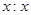is a natural number,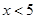and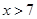}

(iv) {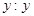is a point common to any two parallel lines}

Ans. (i) Set of odd natural numbers divisible by 2 is an empty set because odd natural numbers are not divisible by 2.

(ii) Set of even prime numbers is {2} which is not empty set.

(iii) {is a natural number,and} is an empty set because there is no natural number which satisfies simultaneouslyand.

(iv) {is a point common to any two parallel lines} is an empty set because two parallel lines do not have a common point.

2. Which of the following sets are finite or infinite:

(i) The set of months of a year.

(ii) {1, 2, 3, ………..}

(iii) {1, 2, 3, ………….., 99, 100}

(iv) The set of positive integers greater than 100.

(v) The set of prime numbers less than 99.

Ans. (i) The set of months of a year is finite set because there are 12 months in a year.

(ii) {1, 2, 3, ………..} is an infinite set because there are infinite elements in the set.

(iii) {1, 2, 3, ………….., 99, 100} is a finite set because the set contains finite number of elements.

(iv) The set of positive integers greater than 100 is an infinite set because there are infinite number of positive integers greater than 100.

(v) The set of prime numbers less than 99 is a finite set because the set contains finite number of elements.

3. State whether each of the following sets is finite or infinite:

(i) The set of lines which are parallel to the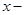axis.

(ii) The set of letters in the English alphabet.

(iii) The set of numbers which are multiple of 5.

(iv) The set of animals living on the earth.

(v) The set of circles passing through the origin (0, 0).

Ans. (i) The set of lines which are parallel to theaxis is an infinite set because we can draw infinite number of lines parallel toaxis.

(ii) The set of letters in the English alphabet is a finite set because there are 26 letters in the English alphabet.

(iii) The set of numbers which are multiple of 5 is an infinite set because there are infinite multiples of 5.

(iv) The set of animals living on the earth is a finite set because the number of animals living on the earth is every large but finite.

(v) The set of circles passing through the origin (0, 0) is an infinite set because we can draw infinite number of circles through origin in different radii.

4. In the following, state whether A = B or not:

(i) A =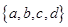B =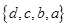(ii) A = {4, 8, 12, 16} B = {8, 4, 16, 18}

(iii) A = {2, 4, 6, 8, 10} B = {is a positive even integer and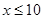}

(iv) A = {is a multiple of 10} B = {10, 15, 20, 25, 30, ….}

Ans. (i) A =and B =are equal sets because order of elements does not change a set. Therefore, A = B =(ii) A = {4, 8, 12, 16} and B = {8, 4, 16, 18} are not equal sets because 12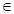A, 12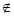B and 18B, 19A

(iii) A = {2, 4, 6, 8, 10} and B = {is a positive even integer and} which can be written in roster form as B = {2, 4, 6, 8, 10} are equal sets.

(iv) A = {is a multiple of 10} can be written in roster form as A = {10, 20, 30, 40, …..} and B = {10, 15, 20, 25, 30, ….} are not equal sets because 15B, 15A.

5. Are the following pairs of sets equal? Give reason.

(i) A = {2, 3} and B = {is a solution of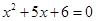}

(ii) A = :is a letter in the word FOLLOW}

B = {is a letter in the word WOLF}

Ans. (i) A = {2, 3} and B

= {is a solution of}

Here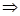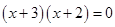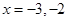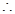B =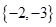Therefore, A and B are not equal sets.

(ii) A = :is a letter in the word FOLLOW} = {F, O, L, W}

B = {is a letter in the word WOLF} = {W, O, L, F}

Therefore, A = B = {F, O, L, W}

6. From the sets given below, select equal sets:

A = {2, 4, 8, 12}

B = {1, 2, 3, 4}

C = {4, 8, 12, 14}

D = {3, 1, 4, 2}

E =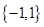F =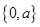G =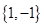H = {0, 1}

Ans. From the given sets, Set B and D have same elements and also sets E and G have same element.1. Mast